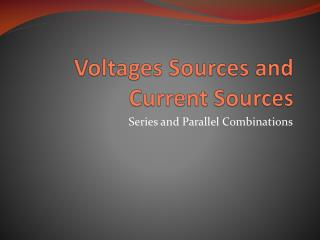# Voltages Sources and Current Sources - PowerPoint PPT PresentationDownload PresentationVoltages Sources and Current Sources

Voltages Sources and Current Sources
Download Presentation## Voltages Sources and Current Sources

- - - - - - - - - - - - - - - - - - - - - - - - - - - E N D - - - - - - - - - - - - - - - - - - - - - - - - - - -
##### Presentation Transcript

1. Voltages Sources and Current Sources Series and Parallel Combinations

2. Objective of Lecture • Explain how voltage sources in series may be combined. • Explain how current sources in parallel may be combined. • Explain under what conditions voltage sources in parallel and current sources in series are allowed.

3. Voltage Sources in Series • DC voltage sources in series can be combined and replaced with a single source. • AC voltage sources in series can be combined and replaced with a single source only if the angular frequency of operation w are identical. • DC and AC voltage sources can be added together when calculating a total voltage. • AC voltage sources operating at different frequencies can be added together. • The current flowing through one voltage source must be equal to the current flowing through the other voltage source.

4. Example 1

5. Example 3 Or Or

6. Example 4 Or Or

7. DC and AC sources • A 5V dc voltage source in series a 2V sin(10t) ac voltage source has a total combined voltage of 5V+2Vsin(10t). • However, we do not have a symbol for a single voltage source that can replace the symbols for the dc and ac sources.

8. AC sources with Different w • A 2V ac voltage source operating at 10 rad/s in series with a 2V ac voltage source operating at 10.5 rad/s has a total voltage of 2Vsin(10t)+2Vsin(10t). • Again, there is not a symbol for a single ac voltage source that can replace the symbols for the two ac sources operating at different frequencies.

9. Voltage Sources in Parallel • Since the voltage sources share common nodes, the only time two or more voltage sources are allowed in parallel is when they have exactly the same voltage, polarity, and frequency of operation (if ac sources). • The multiple voltage sources can be replaced by a single source with the same voltage, polarity, and frequency of operation (if ac sources).

10. Example 5 Allowed Not Allowed

11. Example 6 Allowed Not Allowed

12. Current Sources in Parallel • DC current sources in parallel can be combined and replaced with a single source. • AC current sources in parallel can be combined and replaced with a single source only if the angular frequency of operation w are identical. • DC and AC current sources in parallel can be added together when calculating a total current. • AC current sources operating at different frequencies can be added together. • The voltage drop across one current source must be equal to the voltage dropped across the other current sources in parallel.

13. Example 7

14. Example 8

15. DC and AC Current Sources • A 5A dc current source in parallel a 2A sin(10t) ac current source means that they are contributing a total current of 5A+2Asin(10t) at that node. • However, we do not have a symbol for a single current source that can replace the symbols for the dc and ac sources.

16. AC Sources with Different w • A 2A ac current source operating at 10 rad/s in parallel with a 2V ac current operating at 10.5 rad/s means that they are contributing a total current of 2Asin(10t)+2Asin(10t) at that node. • Again, there is not a symbol for a single ac current source that can replace the symbols for the two ac sources operating at different frequencies.

17. Current Sources in Series • Since components in series must have the same current flowing through each component, the only time two or more currents sources are allowed in series is when they have exactly the same magnitude current, the current is flowing in the same direction, and frequency of operation (if ac sources). • The multiple current sources in series can be replaced by a single source with the same magnitude, direction of current flow, and frequency of operation (if ac sources).

18. Example 10 Allowed Not Allowed

19. Summary • Voltage sources in series can be added. • Current sources in parallel can be added. • Only in the case where the magnitude, polarity, and frequency of operation are identical can multiple voltage sources be in parallel. • They can be replaced with a single voltage source of the same magnitude, polarity, and frequency of operation. • Only in the case where the magnitude, direction of current flow, and frequency of operation are identical can multiple currents sources be in series. • They can be replaced with a single current source of the same magnitude, direction of current flow, and frequency of operation.